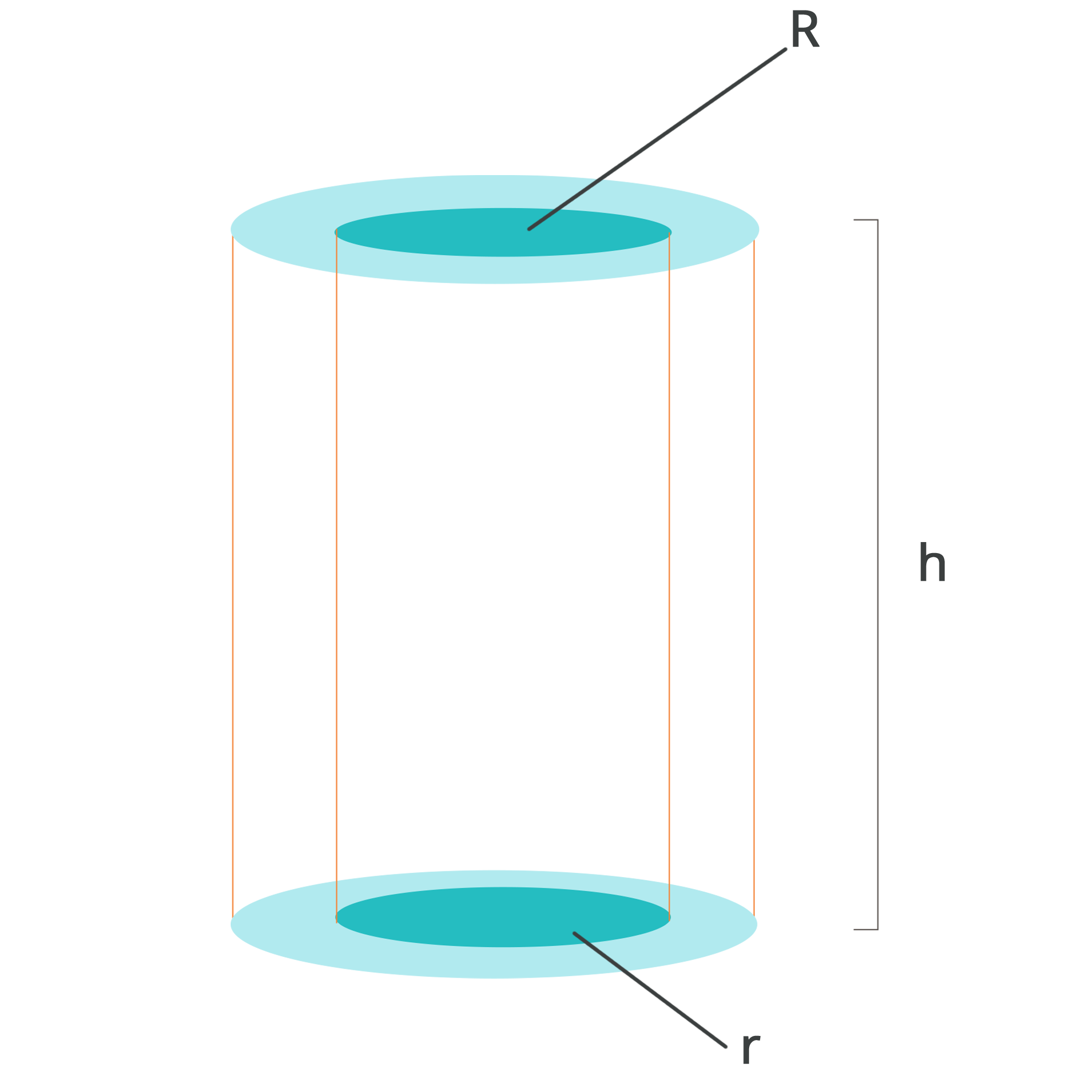• Call Now

1800-102-2727•

# Area of Hollow Cylinder - Maths

Geometry deals with sizes, angles, shapes, dimensions and various other things we witness in our day to day life. These shapes are majorly classified into two types. They are 2-dimensional and 3-dimensional shapes. In-plane geometry, some common shapes such as squares, rectangles, circles, triangles etc., are two-dimensional and flat. On the other hand, in solid geometry, some shapes, namely sphere, cube, cuboid, cone etc., seem to have a three-dimensional structure and are called solid shapes.

A cylinder is one of the most basic 3-dimensional geometric shapes formed by the rotation of a rectangle along any of its sides. It has a curved surface and two circular bases. The curved surface is equidistant from a fixed line segment passing through the centre of its bases. This can be termed the axis of the cylinder. Cylinders find their use in various real-life instances and they are considered very strong load bearing solid shapes which makes their use in bridges and support columns for buildings.A hollow cylinder is considered a form of a cylinder that is vacant on the inside. A hollow pipe is an example of a hollow cylinder where the solid shape contains an inner and an outer radius. A hollow cylinder includes a base which is similar to a circular ring. The solid figure is enclosed between the hollow inner radius and the outer radius. This area of cross section is constant throughout the height ‘h’ of the cylinder therefore, there must be two bases, one on the bottom of the cylinder and the other at the top.

The height of the cylinder can simply be obtained by taking the perpendicular distance between 2 bases. Therefore, the height of the cylinder can also be mentioned as altitude. Meanwhile, the radius of the cylinder is nothing but the radius of the circular base. Real-life examples of hollow cylinders include straws, tubes etc.

## Given below are the formulae for areas of a hollow cylinder,

### i. Total Volume = Volume of External Cylinder – Volume of Internal Cylinder

= πR²h - πr²h

= π(R² - r²)h

One other method of explaining the volume is the area of cross section multiplied by the height. Therefore in the case of a hollow sphere the volume can be found by multiplying the area of the ring-like circular base with its height.

= 2πRh + 2πrh

= 2πh(R + r)

### iii. Total Surface Area = Lateral Surface Area + Areas of Solid Bases

2π(R + r) + 2π(R² - r²)

Where,

π=22/7 or 3.14

Surface area is a simple measurement of all the exposed areas of a solid object. is the symbol of the mathematical term pi. Pi is determined by the ratio of a circle’s circumference to its diameter. Furthermore, hollow cylinders weigh less than those solid ones in real-time applications. This is because of the absence of inner materials inside a hollow object. So, considering the absence of material, the strength of hollow objects is also low when compared to solid ones.Talk to our expert
Resend OTP Timer =
By submitting up, I agree to receive all the Whatsapp communication on my registered number and Aakash terms and conditions and privacy policy